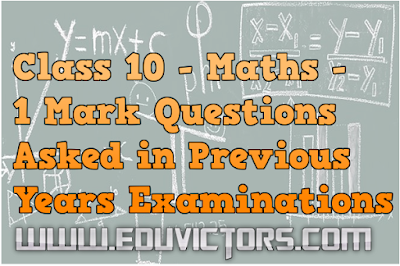## Class 10 - Maths - 1 Mark Questions Asked in Previous Years Examinations

Q1: If secθ.sinθ = 0, find tan θ.

secθ.sinθ = tan θ = 0
⇛ tan θ = tan 0°
∴ θ = 0°

Q2: If tan(3x + 30°) = 1, find the value of x

tan(3x + 30°) = 1 = tan 45°
3x + 30° = 45°
3x = 45 - 30 = 15
x = 5°

Q3: When are two triangles said to be similar?

Answer: Two triangles are said to be similar when corresponding sides are proportional and angles are equal.

Q4: To divide the line segment AB in the ratio 2:3, a ray AX is drawn such that ∠BAX is acute, AX is marked at equal intervals. Find the minimum number of such marks.

Answer: Minimum no. of marks = 2 + 3 = 5.

Q5: What is the maximum number of parallel tangents a circle can have on a diameter?

Q6: Are two triangles with equal corresponding sides always similar?

Answer: No, included angles must also be equal.

Q7: Find the distance of the point (-4, -7) from y-axis?

Answer: 4 units (The x-coordinate of point is -4)
Using distance formula,
```
___________________    ___________
Distance = √(0 + 4)² + (7 - 7)² = √(4)² + (0)²
```

= √16 = 4 units

Q8: Find the sum of first five multiples of 2.

Answer: Here a = 2, d = 2, n = 5
S = n[2a + (n-1)d]/2
= 5[2(2) + (5-1)2]/2 = 5 × 6
= 30

Q9: In the AP series three numbers are 2, x, 26, find the value of x.

Answer: Common difference will be equal.
x - 2 = 26 - x
x + x = 26 + 2
2x = 28
x = 14

______
```Q10: Find the positive root of √3x² + 6  = 9
_______
Answer: √3x² + 6  = 9

(3x² + 6)  = 9²
⇛   3x² = 81 - 6 = 75
⇛    x² = 25
⇛  x = 5
```

Q11: If α and β are the roots of ax² -bx + c = 0 (a ≠ 0). Find α + β.

Answer: Sum of roots = α + β = -b/a = -(-b/a) = b/a

Q12: Explain why 13233354363715 is a composite number?

Answer: Since the given number ends with digit 5, it is a multiple of 5. ∴ it is a composite number.